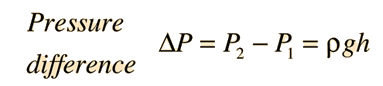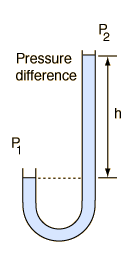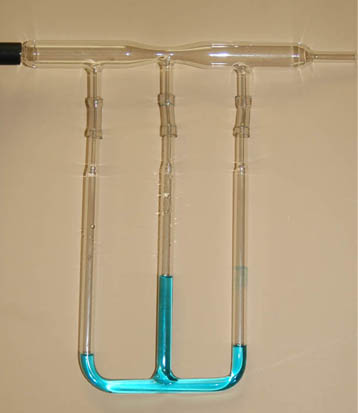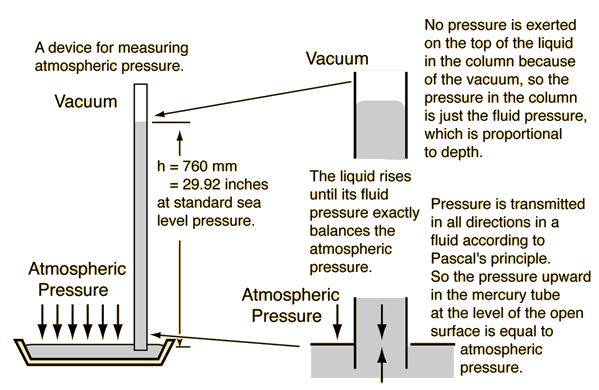Fluid Pressure Measurement

 Since static fluid pressure is determined by the fluid density and depth, the depth or height difference of a given liquid is commonly used for pressure measurement:The fact that the liquid levels are the same in these three manometers shows that the pressure in the glass manifold above them is uniform. This is under static conditions with no air flow through the system so that all parts of it are at atmospheric pressure. The fact that open liquids will seek a common level is the principle behind liquid levels for construction purposes.
 The flow in this system is from left to right, driven by a high pressure air supply. The system is said to have a positive gauge pressure exerted by the air supply. This pressure acts to push the manometer levels down, so the minimum height in the left manometer indicates that it is subjected to the highest pressure.The fact that the center manometer has a higher level under these conditions of rapid air flow indicates that the pressure has been lowered in the constriction by the Bernoulli effect. Note that the liquid level in the right hand tube is slightly higher than the left tube, indicating that the pressure there is slightly less than that at the left hand tube. This is indicative of the normal pressure drop in a flowing fluid from Poiseuille's law since the diameters of the top tube are the same at the left and right tubes.

Pressure Calculation

Index

Pressure concepts

 HyperPhysics***** Mechanics ***** Fluids R Nave
Go Back

Fluid Pressure CalculationDiscussion

Fluid column height in the relationshipis often used for the measurement of pressure. After entering the relevant data, any one of the highlighted quantities below can be calculated by clicking on it.

Pressure difference = density x g x height

If the fluid density is

ρ = gm/cm3 = kg/m3

and the column height is

h = m = x 10^ m
h = ft

then the pressure difference is

ΔP = kPa
ΔP = lb/in2
ΔP = mmHg= inches Hg
ΔP = atmos
ΔP = inches water= cm water
Index

Pressure concepts

 HyperPhysics***** Mechanics ***** Fluids R Nave
Go Back

Atmospheric Pressure

The surface of the earth is at the bottom of an atmospheric sea. The standard atmospheric pressure is measured in various units:

1 atmosphere = 760 mmHg = 29.92 inHg = 14.7 lb/in2 = 101.3 KPa

The fundamental SI unit of pressure is the Pascal (Pa), but it is a small unit so kPa is the most common direct pressure unit for atmospheric pressure. Since the static fluid pressure is dependent only upon density and depth, choosing a liquid of standard density like mercury or water allows you to express the pressure in units of height or depth, e.g., mmHg or inches of water. The mercury barometer is the standard instrument for atmospheric pressure measurement in weather reporting. The decrease in atmospheric pressure with height can be predicted from the barometric formula.

The unit mmHg is often called torr, particularly in vacuum applications: 760 mmHg = 760 torr

For weather applications, the standard atmospheric pressure is often called 1 bar or 1000 millibars. This has been found to be convenient for recording the relatively small deviations from standard atmospheric pressure with normal weather patterns.

 Force of atmosphere on an area.
 Constituents of the atmosphere
 Standard temperature and pressure (STP)
Index

Atmospheric pressure demos

Pressure concepts

 HyperPhysics***** Mechanics ***** Fluids R Nave
Go Back

Mercury Barometer

The mercury barometer and other manometer devices are reliable pressure measurement devices since fluid pressure depends only on fluid density and depth. The atmospheric pressure provides the force necessary to push the mercury up the evacuated tube. Even though it acts downward in the illustration, by Pascal's principle we know that the pressure acts in all directions and can force the mercury up the tube until its weight is enough to equal the force of the atmosphere.Index

Pressure concepts

 HyperPhysics***** Mechanics ***** Fluids R Nave
Go Back